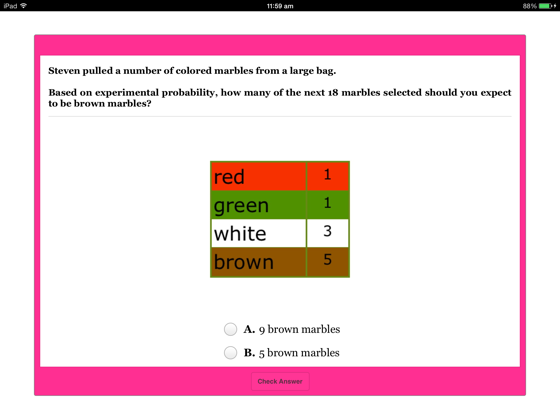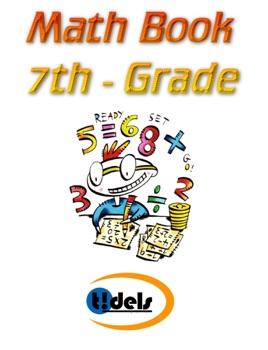## Publisher Description

Tidels Math Book Grade 7 focuses on Ratios & Proportions, Pythagorean theorem, Percentage, Linear Function, Properties, Measurements, Consumer Math, Probability. This e-book includes a collection of 200 math problems in the 7 categories. Students aged above 12 years can enjoy doing the problems.

Tidels brings you Math learning Workbook for your children to learn 7th Grade Math Lessons.  The Complete Book of Math provides 200 pages of fun exercises for students in Grade 7 that teach students key lessons in math.

Learning the fundamentals of mathematics during the early years is crucial. Math Book helps your children in a  great way to practice the basics of mathematics. When children genuinely understand the basics of mathematics, they will have the confidence and capability to appreciate more advanced mathematics. Six to Eight year children will love these colorful and imaginative book. Our Math books are intended to enhance your child's skills and introduce new concepts in a fun-filled  and stress-free manner.

••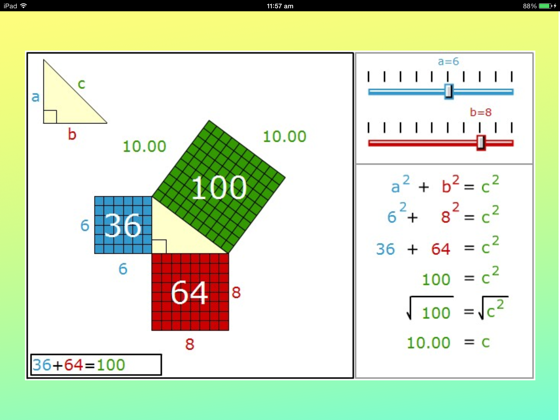•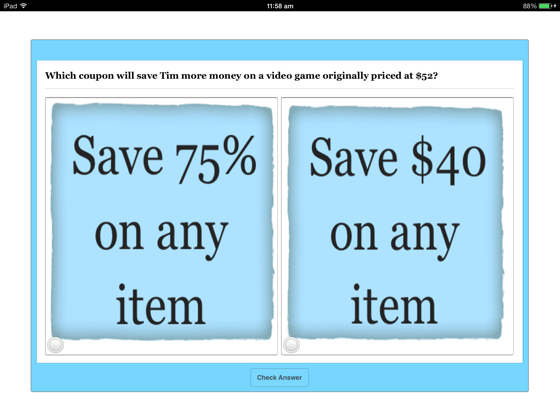•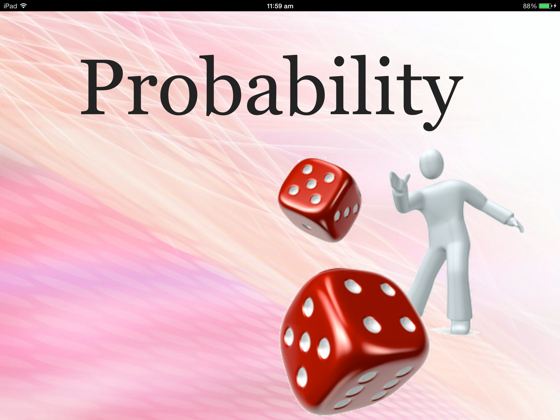•